# SummaryType Enum

Lists values that specify the summary function types.

Namespace: DevExpress.DashboardCommon

Assembly: DevExpress.Dashboard.v23.1.Core.dll

NuGet Packages: DevExpress.Dashboard.Core, DevExpress.Win.Dashboard.Design

## Declaration

``public enum SummaryType``

## Members

Name Description
`Count`

The number of values (excluding Null and DBNull values).

`Sum`

The sum of the values.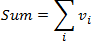`Min`

The smallest value.

`Max`

The largest value.

`Average`

The average of the values.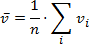`StdDev`

An estimate of the standard deviation of a population, where the sample is a subset of the entire population.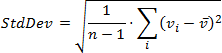`StdDevp`

The standard deviation of a population, where the population is all of the data to be summarized.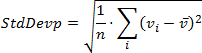`Var`

An estimate of the variance of a population, where the sample is a subset of the entire population.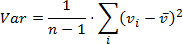`Varp`

The variance of a population, where the population is all of the data to be summarized.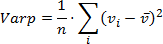`CountDistinct`

The number of distinct values.

`Median`

The median of the values. A median is the number separating the higher half of a value range from the lower half.

`Mode`

The mode of the values. A mode is the value that is repeated more often than any other.

## Remarks

Values listed by this enumeration are used to set the Measure.SummaryType property.